Скачать презентацию LEXICALIZATION AND CATEGORIAL GRAMMARS A STORY BAR-HILLEL MIGHT

da6946acff3b783130525567cc4ec837.ppt

• Количество слайдов: 54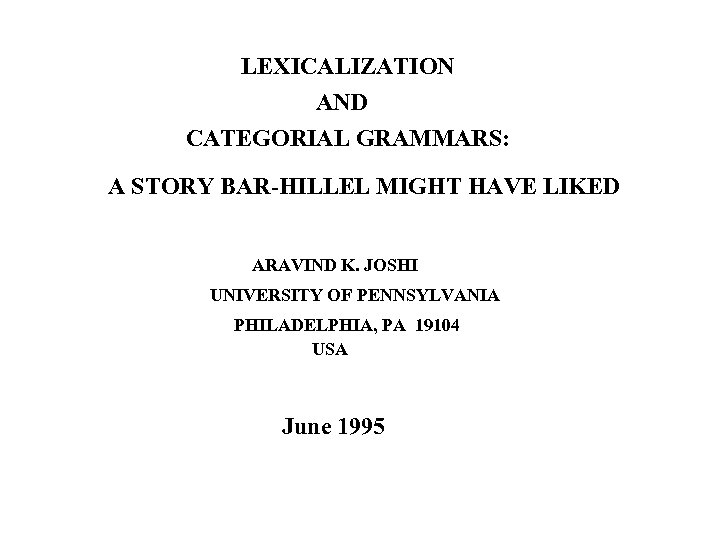LEXICALIZATION AND CATEGORIAL GRAMMARS: A STORY BAR-HILLEL MIGHT HAVE LIKED ARAVIND K. JOSHI UNIVERSITY OF PENNSYLVANIA PHILADELPHIA, PA 19104 USA June 1995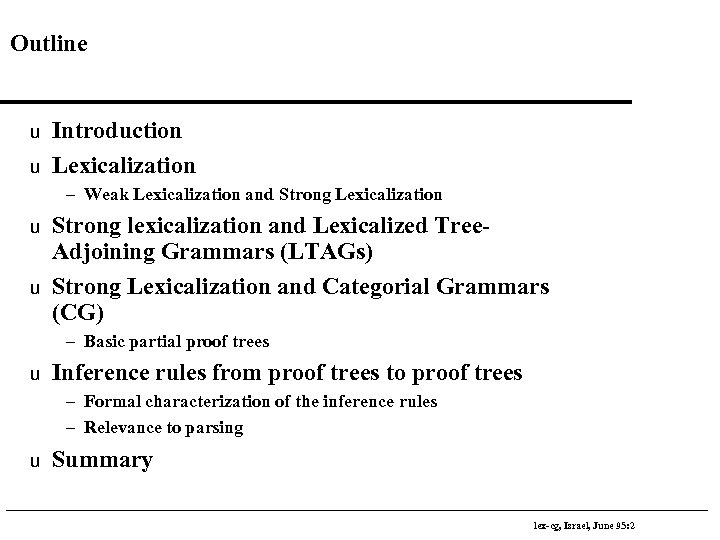Outline u u Introduction Lexicalization – Weak Lexicalization and Strong Lexicalization u u Strong lexicalization and Lexicalized Tree. Adjoining Grammars (LTAGs) Strong Lexicalization and Categorial Grammars (CG) – Basic partial proof trees u Inference rules from proof trees to proof trees – Formal characterization of the inference rules – Relevance to parsing u Summary lex-cg, Israel, June 95: 2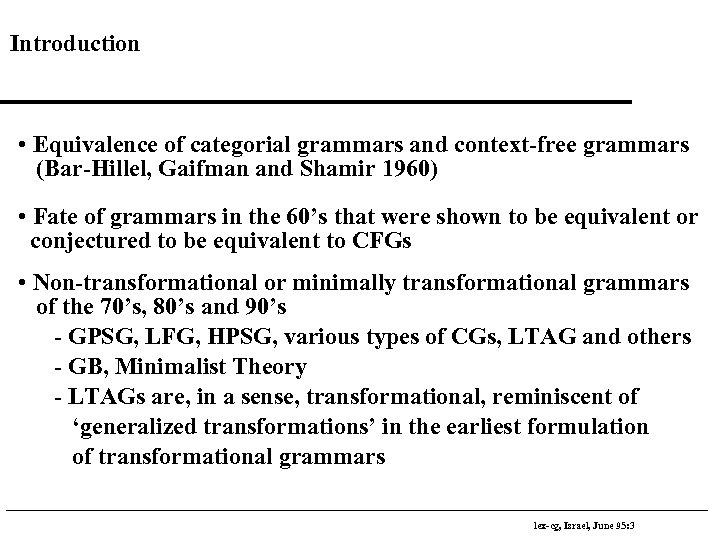Introduction • Equivalence of categorial grammars and context-free grammars (Bar-Hillel, Gaifman and Shamir 1960) • Fate of grammars in the 60’s that were shown to be equivalent or conjectured to be equivalent to CFGs • Non-transformational or minimally transformational grammars of the 70’s, 80’s and 90’s - GPSG, LFG, HPSG, various types of CGs, LTAG and others - GB, Minimalist Theory - LTAGs are, in a sense, transformational, reminiscent of ‘generalized transformations’ in the earliest formulation of transformational grammars lex-cg, Israel, June 95: 3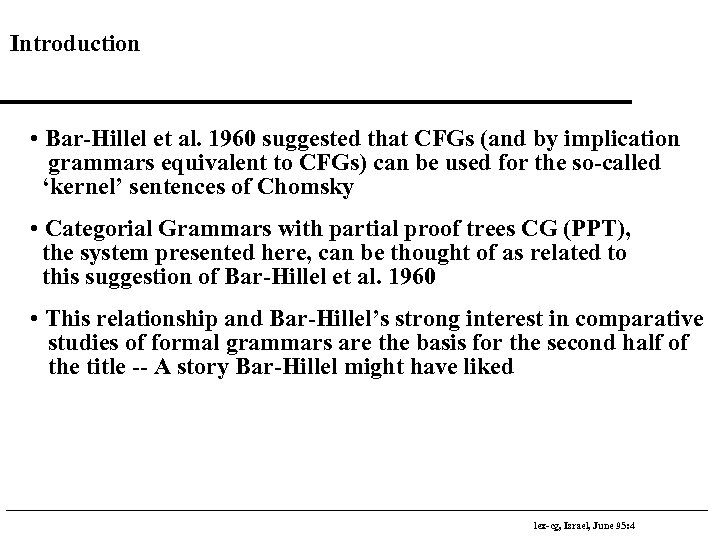Introduction • Bar-Hillel et al. 1960 suggested that CFGs (and by implication grammars equivalent to CFGs) can be used for the so-called ‘kernel’ sentences of Chomsky • Categorial Grammars with partial proof trees CG (PPT), the system presented here, can be thought of as related to this suggestion of Bar-Hillel et al. 1960 • This relationship and Bar-Hillel’s strong interest in comparative studies of formal grammars are the basis for the second half of the title -- A story Bar-Hillel might have liked lex-cg, Israel, June 95: 4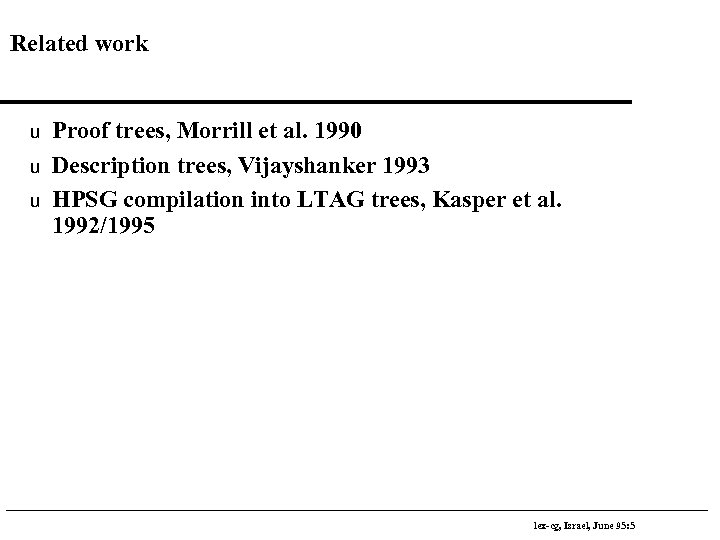Related work u u u Proof trees, Morrill et al. 1990 Description trees, Vijayshanker 1993 HPSG compilation into LTAG trees, Kasper et al. 1992/1995 lex-cg, Israel, June 95: 5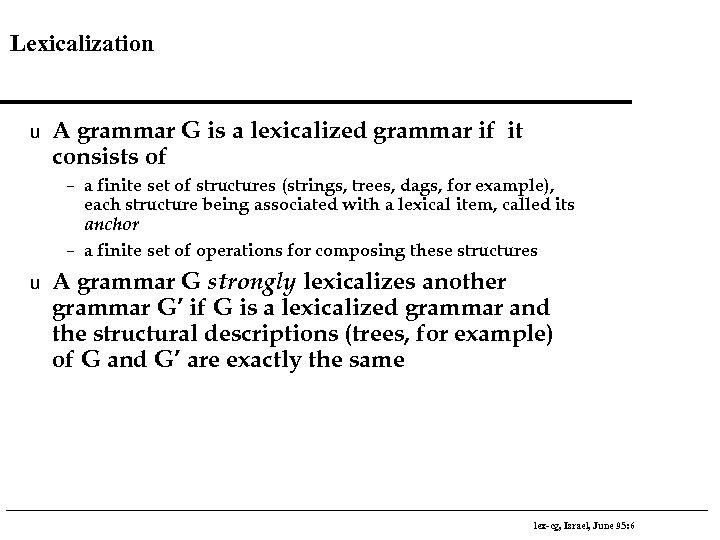Lexicalization u A grammar G is a lexicalized grammar if it consists of – a finite set of structures (strings, trees, dags, for example), each structure being associated with a lexical item, called its anchor – a finite set of operations for composing these structures u A grammar G strongly lexicalizes another grammar G’ if G is a lexicalized grammar and the structural descriptions (trees, for example) of G and G’ are exactly the same lex-cg, Israel, June 95: 6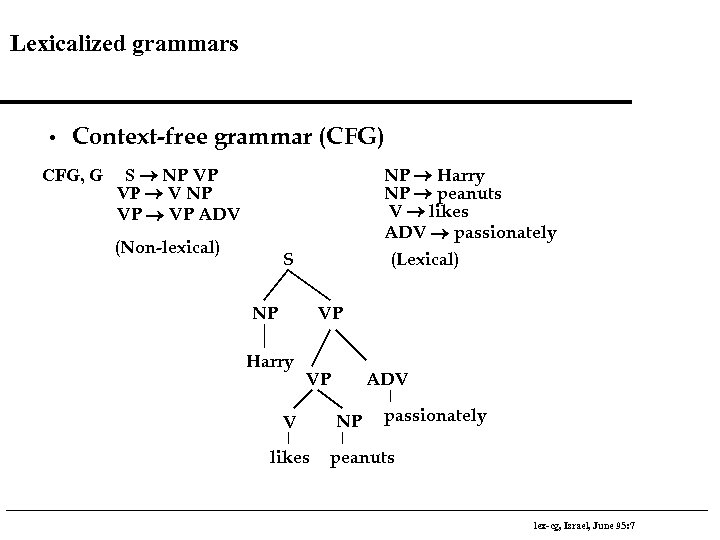Lexicalized grammars • Context-free grammar (CFG) CFG, G S ® NP VP VP ® V NP VP ® VP ADV (Non-lexical) NP ® Harry NP ® peanuts V ® likes ADV ® passionately (Lexical) S NP VP Harry VP V likes ADV NP passionately peanuts lex-cg, Israel, June 95: 7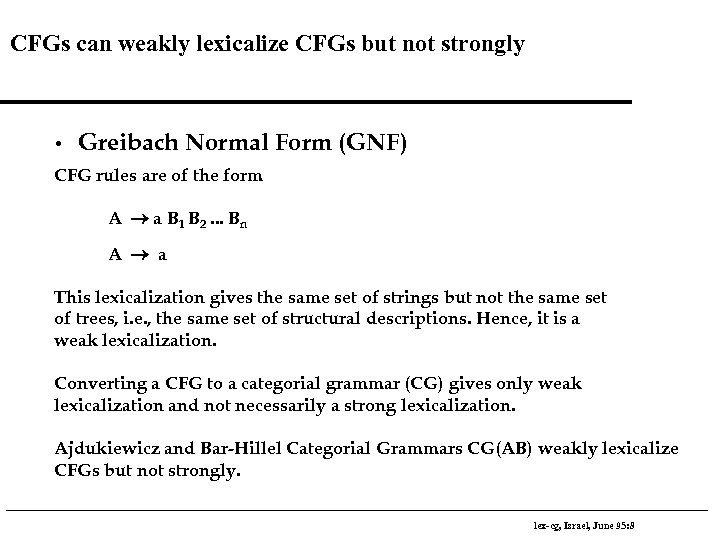CFGs can weakly lexicalize CFGs but not strongly • Greibach Normal Form (GNF) CFG rules are of the form A ® a B 1 B 2. . . Bn A ® a This lexicalization gives the same set of strings but not the same set of trees, i. e. , the same set of structural descriptions. Hence, it is a weak lexicalization. Converting a CFG to a categorial grammar (CG) gives only weak lexicalization and not necessarily a strong lexicalization. Ajdukiewicz and Bar-Hillel Categorial Grammars CG(AB) weakly lexicalize CFGs but not strongly. lex-cg, Israel, June 95: 8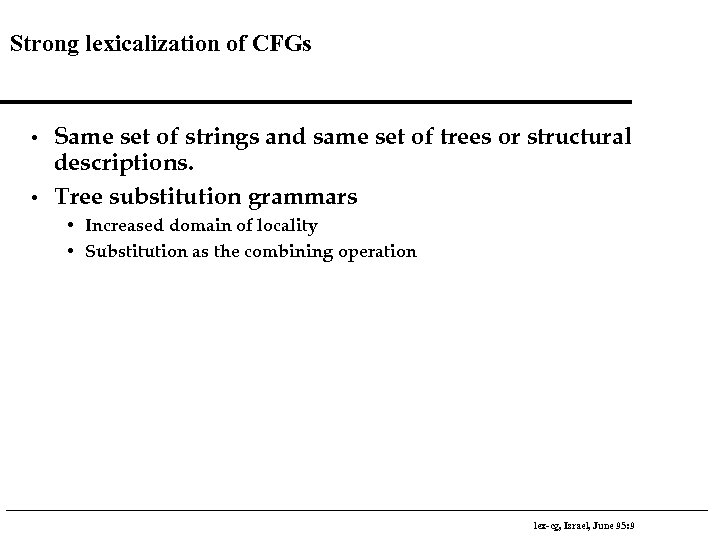Strong lexicalization of CFGs • • Same set of strings and same set of trees or structural descriptions. Tree substitution grammars • Increased domain of locality • Substitution as the combining operation lex-cg, Israel, June 95: 9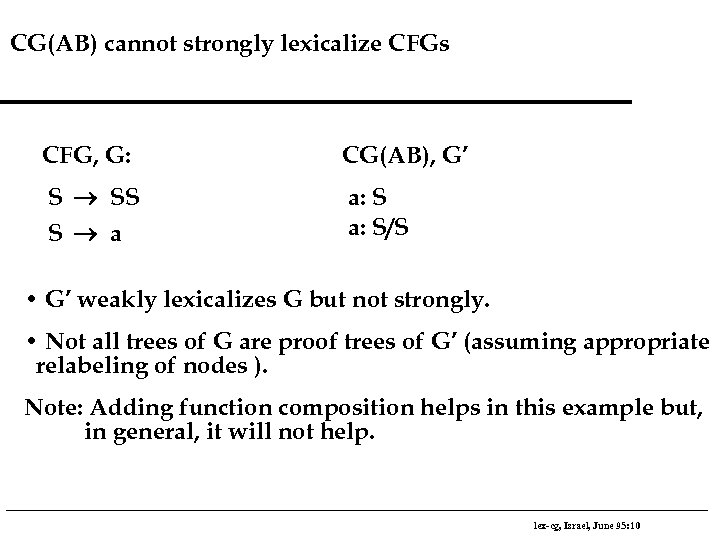CG(AB) cannot strongly lexicalize CFGs CFG, G: CG(AB), G’ S ® SS S ® a a: S/S • G’ weakly lexicalizes G but not strongly. • Not all trees of G are proof trees of G’ (assuming appropriate relabeling of nodes ). Note: Adding function composition helps in this example but, in general, it will not help. lex-cg, Israel, June 95: 10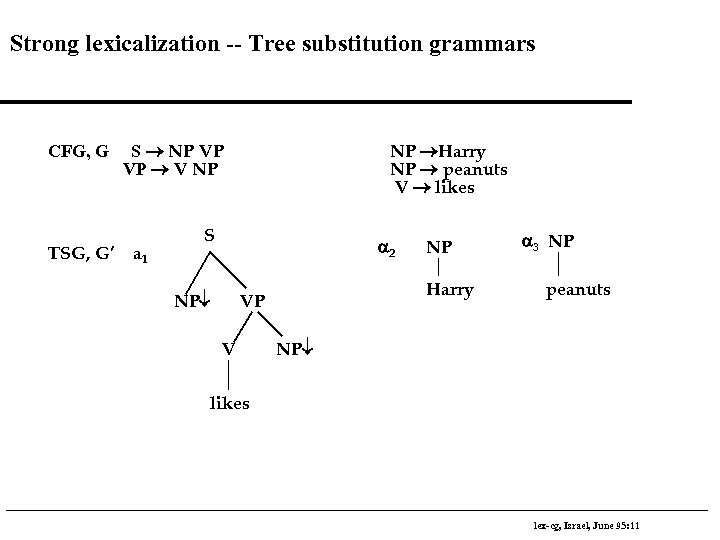Strong lexicalization -- Tree substitution grammars CFG, G S ® NP VP VP ® V NP TSG, G’ a 1 NP ®Harry NP ® peanuts V ® likes S a 2 NP¯ Harry VP V NP a 3 NP peanuts NP¯ likes lex-cg, Israel, June 95: 11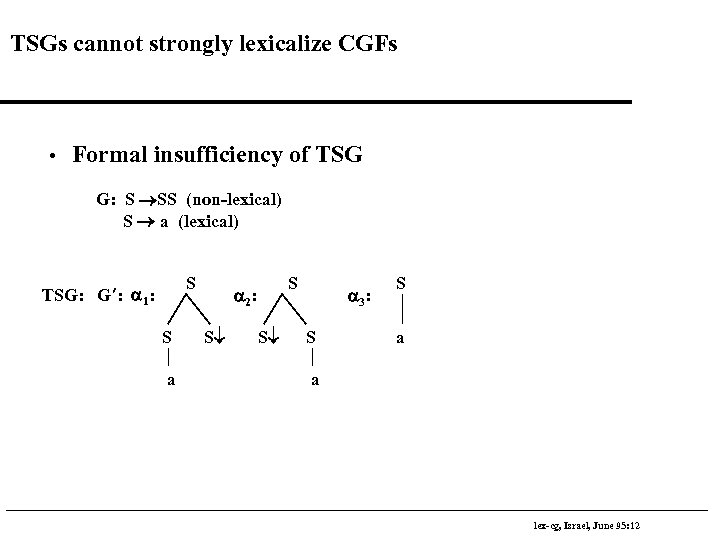TSGs cannot strongly lexicalize CGFs • Formal insufficiency of TSG G: S ®SS (non-lexical) S ® a (lexical) S TSG: G’: a 1: S a 2 : S¯ S¯ a 3 : S S a a lex-cg, Israel, June 95: 12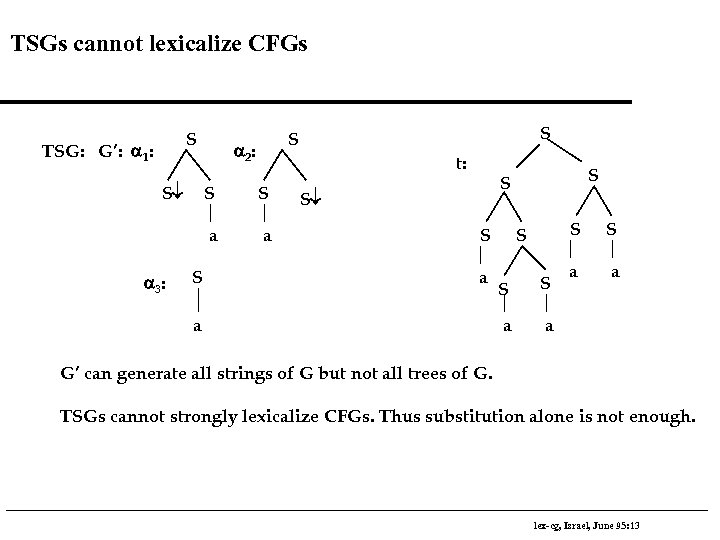TSGs cannot lexicalize CFGs S TSG: G’: a 1: S¯ a 2 : t: S S S a a 3 : S S a S S S¯ S a a S S a S a a a G’ can generate all strings of G but not all trees of G. TSGs cannot strongly lexicalize CFGs. Thus substitution alone is not enough. lex-cg, Israel, June 95: 13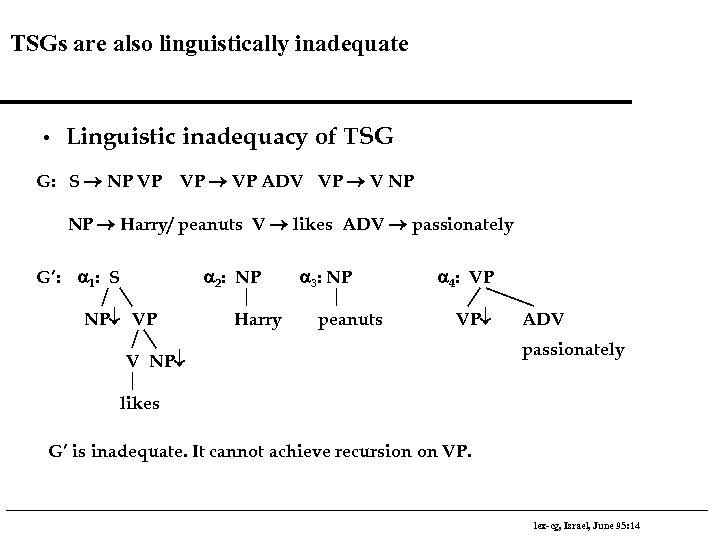TSGs are also linguistically inadequate • Linguistic inadequacy of TSG G: S ® NP VP VP ® VP ADV VP ® V NP NP ® Harry/ peanuts V ® likes ADV ® passionately G’: a 1: S a 2: NP NP¯ VP Harry a 3: NP peanuts a 4: VP VP¯ V NP¯ ADV passionately likes G’ is inadequate. It cannot achieve recursion on VP. lex-cg, Israel, June 95: 14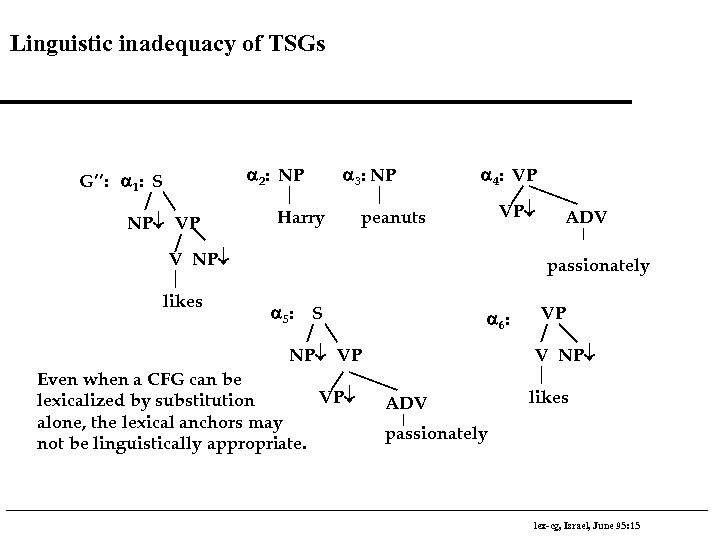Linguistic inadequacy of TSGs a 2: NP G’’: a 1: S NP¯ VP a 3: NP Harry a 4: VP VP¯ peanuts V NP¯ likes ADV passionately a 5 : S a 6 : NP¯ VP Even when a CFG can be VP¯ lexicalized by substitution alone, the lexical anchors may not be linguistically appropriate. VP V NP¯ ADV likes passionately lex-cg, Israel, June 95: 15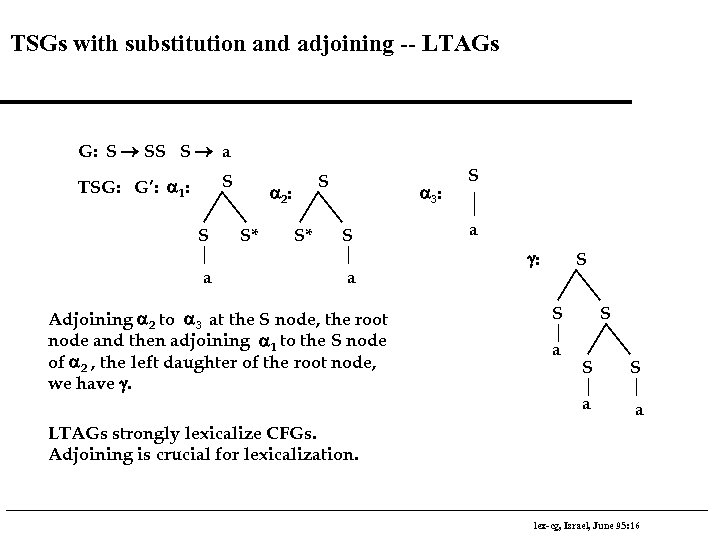TSGs with substitution and adjoining -- LTAGs G: S ® SS S ® a S TSG: G’: a 1: S a 2 : S* S* a 3 : S a Adjoining a 2 to a 3 at the S node, the root node and then adjoining a 1 to the S node of a 2 , the left daughter of the root node, we have g. S a g: S S a S S S a a LTAGs strongly lexicalize CFGs. Adjoining is crucial for lexicalization. lex-cg, Israel, June 95: 16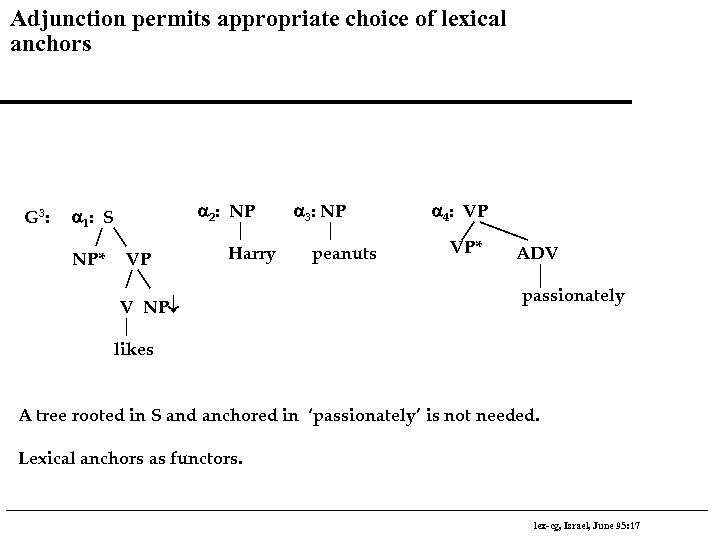Adjunction permits appropriate choice of lexical anchors G 3 : a 2: NP a 1 : S NP* VP Harry V NP¯ a 3: NP peanuts a 4: VP VP* ADV passionately likes A tree rooted in S and anchored in ‘passionately’ is not needed. Lexical anchors as functors. lex-cg, Israel, June 95: 17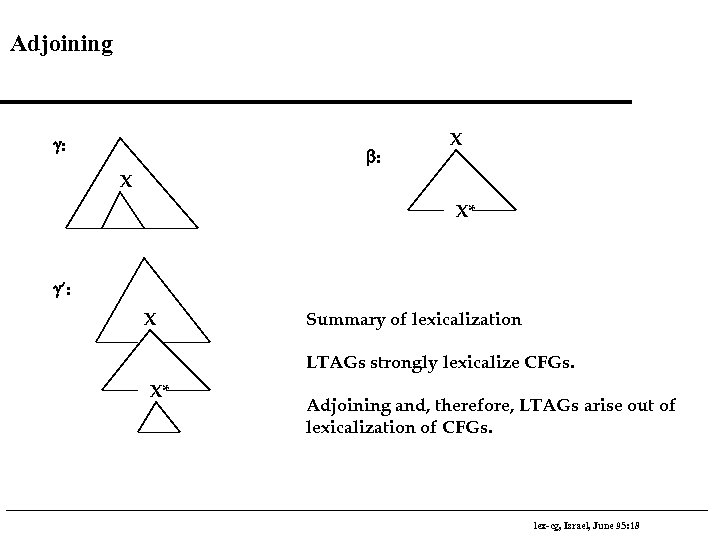Adjoining g: b: X X X* g’: X Summary of lexicalization LTAGs strongly lexicalize CFGs. X* Adjoining and, therefore, LTAGs arise out of lexicalization of CFGs. lex-cg, Israel, June 95: 18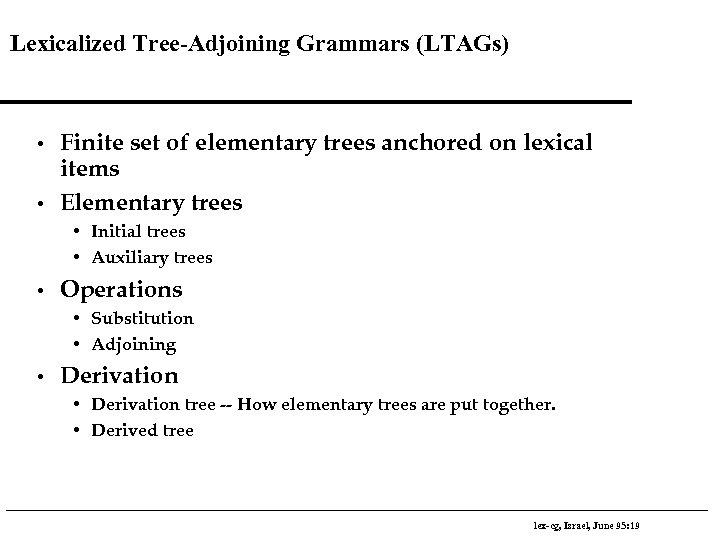Lexicalized Tree-Adjoining Grammars (LTAGs) • • Finite set of elementary trees anchored on lexical items Elementary trees • Initial trees • Auxiliary trees • Operations • Substitution • Adjoining • Derivation • Derivation tree -- How elementary trees are put together. • Derived tree lex-cg, Israel, June 95: 19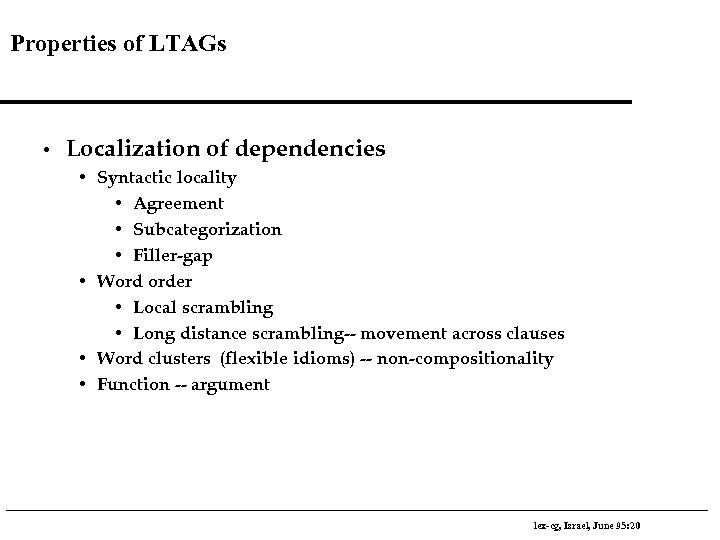Properties of LTAGs • Localization of dependencies • Syntactic locality • Agreement • Subcategorization • Filler-gap • Word order • Local scrambling • Long distance scrambling-- movement across clauses • Word clusters (flexible idioms) -- non-compositionality • Function -- argument lex-cg, Israel, June 95: 20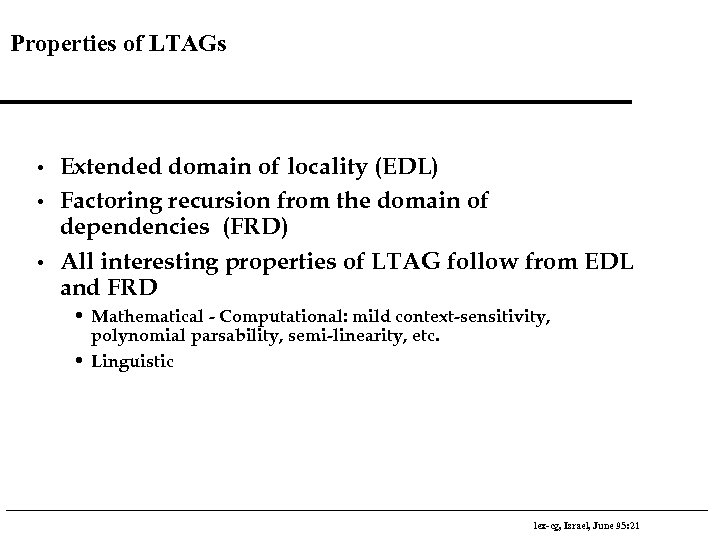Properties of LTAGs • • • Extended domain of locality (EDL) Factoring recursion from the domain of dependencies (FRD) All interesting properties of LTAG follow from EDL and FRD • Mathematical - Computational: mild context-sensitivity, polynomial parsability, semi-linearity, etc. • Linguistic lex-cg, Israel, June 95: 21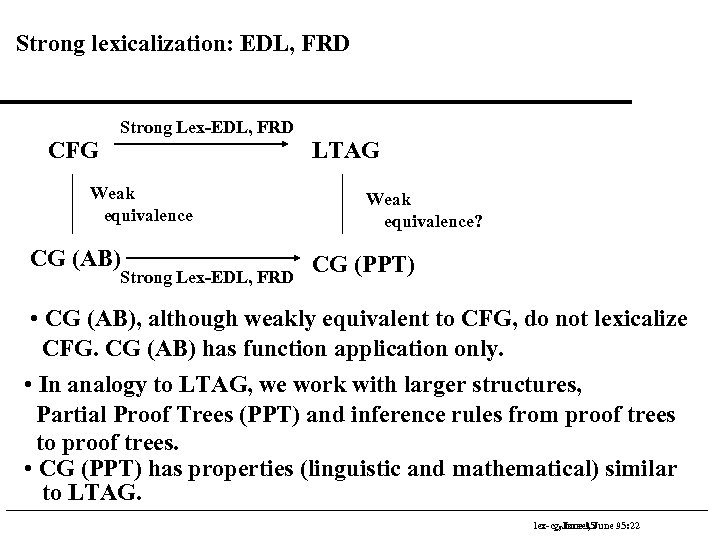Strong lexicalization: EDL, FRD CFG Strong Lex-EDL, FRD Weak equivalence CG (AB) Strong Lex-EDL, FRD LTAG Weak equivalence? CG (PPT) • CG (AB), although weakly equivalent to CFG, do not lexicalize CFG. CG (AB) has function application only. • In analogy to LTAG, we work with larger structures, Partial Proof Trees (PPT) and inference rules from proof trees to proof trees. • CG (PPT) has properties (linguistic and mathematical) similar to LTAG. lex-cg-June 95 lex-cg, Israel, June 95: 22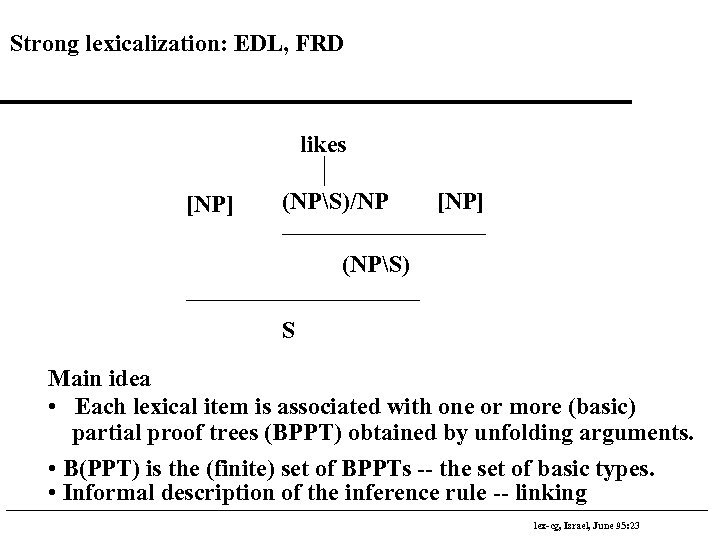Strong lexicalization: EDL, FRD likes [NP] (NPS)/NP [NP] (NPS) S Main idea • Each lexical item is associated with one or more (basic) partial proof trees (BPPT) obtained by unfolding arguments. • B(PPT) is the (finite) set of BPPTs -- the set of basic types. • Informal description of the inference rule -- linking lex-cg, Israel, June 95: 23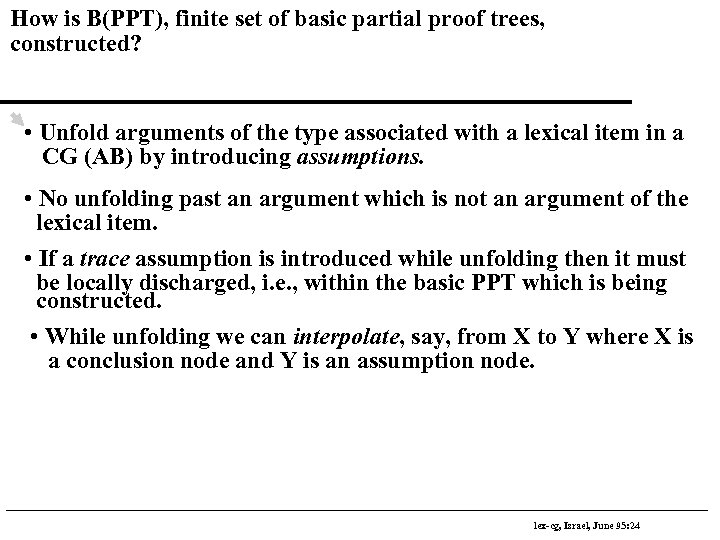How is B(PPT), finite set of basic partial proof trees, constructed? • Unfold arguments of the type associated with a lexical item in a CG (AB) by introducing assumptions. • No unfolding past an argument which is not an argument of the lexical item. • If a trace assumption is introduced while unfolding then it must be locally discharged, i. e. , within the basic PPT which is being constructed. • While unfolding we can interpolate, say, from X to Y where X is a conclusion node and Y is an assumption node. lex-cg, Israel, June 95: 24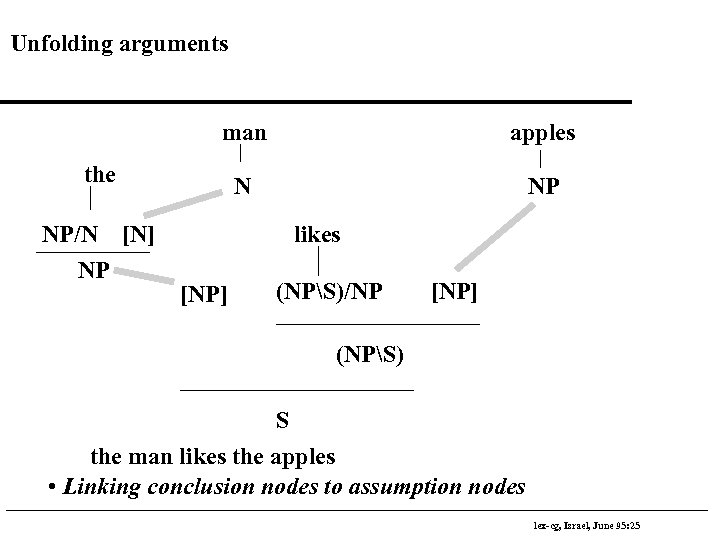Unfolding arguments man apples N NP the NP/N [N] NP likes [NP] (NPS)/NP [NP] (NPS) S the man likes the apples • Linking conclusion nodes to assumption nodes lex-cg, Israel, June 95: 25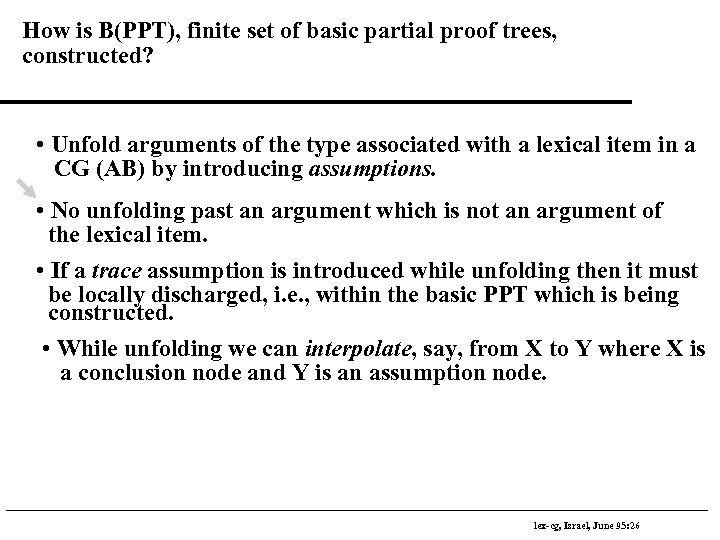How is B(PPT), finite set of basic partial proof trees, constructed? • Unfold arguments of the type associated with a lexical item in a CG (AB) by introducing assumptions. • No unfolding past an argument which is not an argument of the lexical item. • If a trace assumption is introduced while unfolding then it must be locally discharged, i. e. , within the basic PPT which is being constructed. • While unfolding we can interpolate, say, from X to Y where X is a conclusion node and Y is an assumption node. lex-cg, Israel, June 95: 26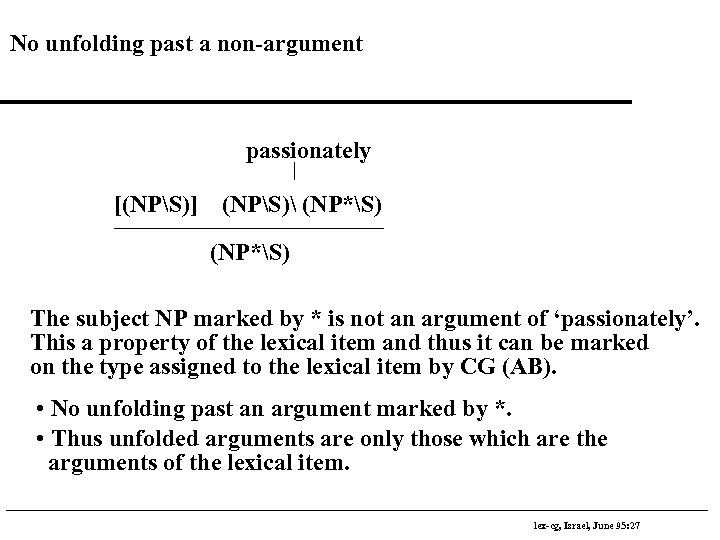No unfolding past a non-argument passionately [(NPS)] (NPS) (NP*S) The subject NP marked by * is not an argument of ‘passionately’. This a property of the lexical item and thus it can be marked on the type assigned to the lexical item by CG (AB). • No unfolding past an argument marked by *. • Thus unfolded arguments are only those which are the arguments of the lexical item. lex-cg, Israel, June 95: 27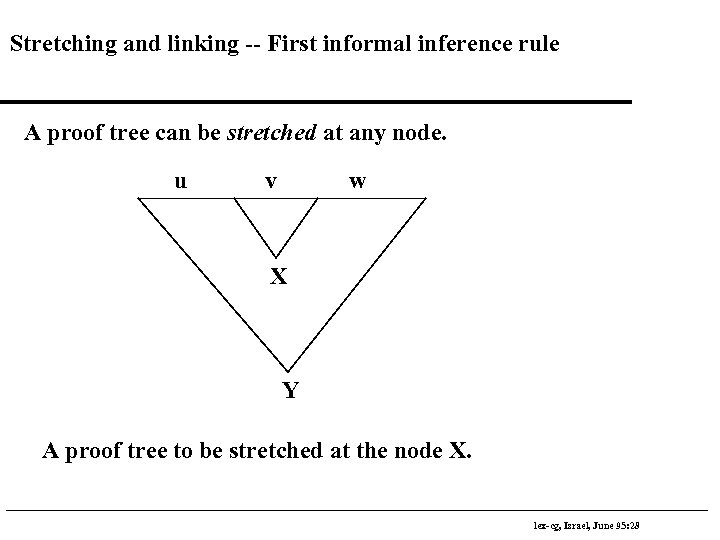Stretching and linking -- First informal inference rule A proof tree can be stretched at any node. u v w X Y A proof tree to be stretched at the node X. lex-cg, Israel, June 95: 28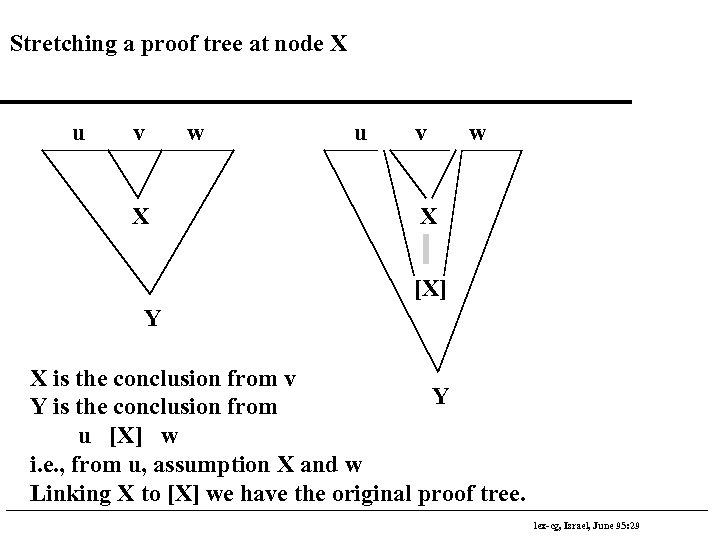Stretching a proof tree at node X u v X w u v w X [X] Y X is the conclusion from v Y Y is the conclusion from u [X] w i. e. , from u, assumption X and w Linking X to [X] we have the original proof tree. lex-cg, Israel, June 95: 29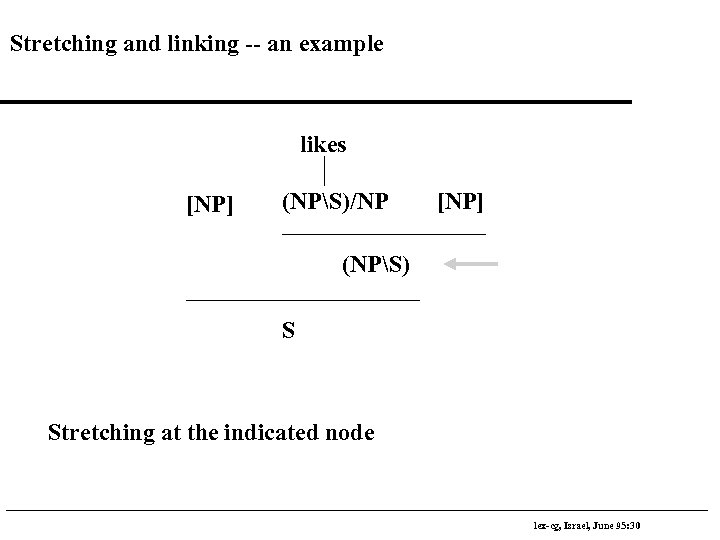Stretching and linking -- an example likes [NP] (NPS)/NP [NP] (NPS) S Stretching at the indicated node lex-cg, Israel, June 95: 30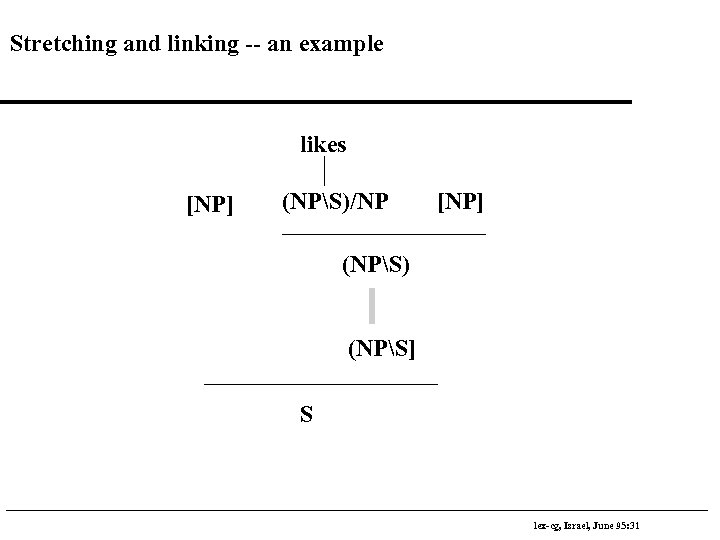Stretching and linking -- an example likes [NP] (NPS)/NP [NP] (NPS) (NPS] S lex-cg, Israel, June 95: 31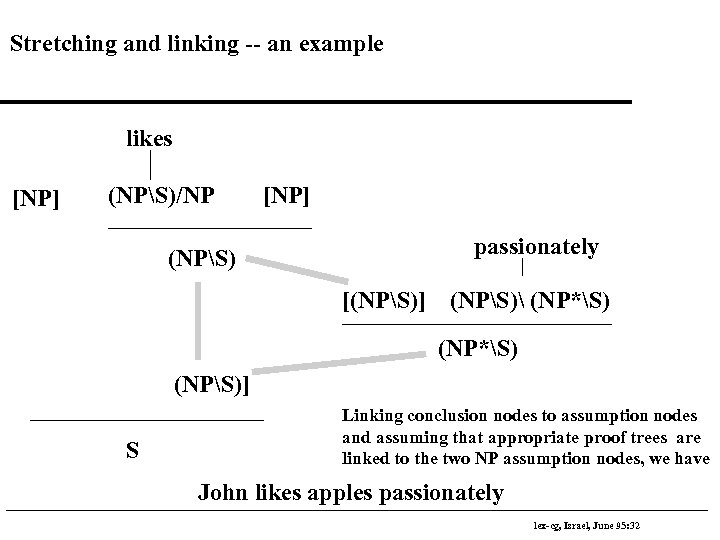Stretching and linking -- an example likes [NP] (NPS)/NP (NPS) [NP] passionately [(NPS)] (NPS) (NP*S) (NPS)] S Linking conclusion nodes to assumption nodes and assuming that appropriate proof trees are linked to the two NP assumption nodes, we have John likes apples passionately lex-cg, Israel, June 95: 32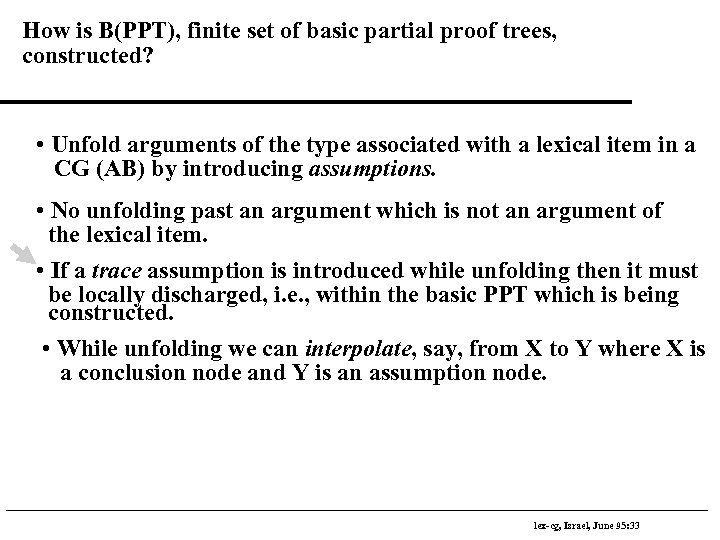How is B(PPT), finite set of basic partial proof trees, constructed? • Unfold arguments of the type associated with a lexical item in a CG (AB) by introducing assumptions. • No unfolding past an argument which is not an argument of the lexical item. • If a trace assumption is introduced while unfolding then it must be locally discharged, i. e. , within the basic PPT which is being constructed. • While unfolding we can interpolate, say, from X to Y where X is a conclusion node and Y is an assumption node. lex-cg, Israel, June 95: 33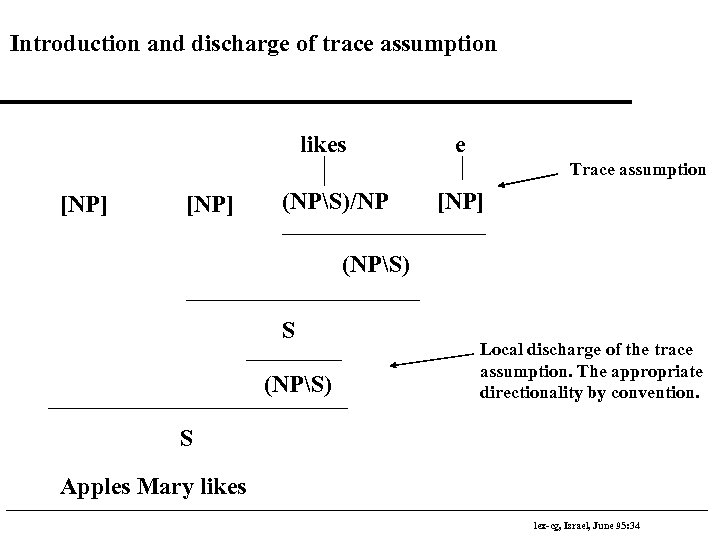Introduction and discharge of trace assumption likes e Trace assumption [NP] (NPS)/NP [NP] (NPS) S (NPS) Local discharge of the trace assumption. The appropriate directionality by convention. S Apples Mary likes lex-cg, Israel, June 95: 34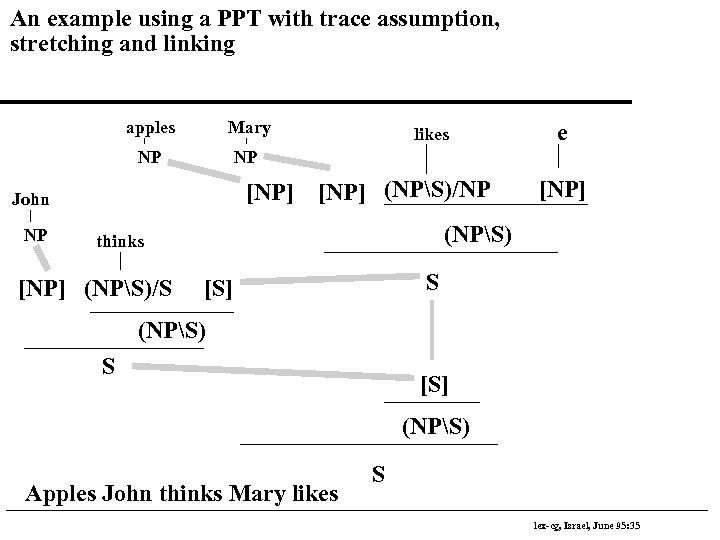An example using a PPT with trace assumption, stretching and linking apples Mary NP NP [NP] John NP likes [NP] (NPS)/NP [NP] (NPS) thinks [NP] (NPS)/S e S [S] (NPS) Apples John thinks Mary likes S lex-cg, Israel, June 95: 35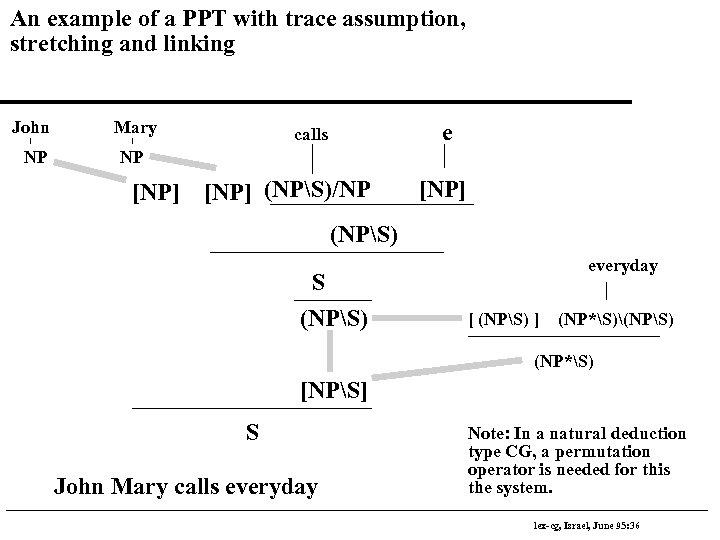An example of a PPT with trace assumption, stretching and linking John Mary NP NP [NP] e calls [NP] (NPS)/NP [NP] (NPS) S (NPS) everyday [ (NPS) ] (NP*S)(NPS) (NP*S) [NPS] S John Mary calls everyday Note: In a natural deduction type CG, a permutation operator is needed for this the system. lex-cg, Israel, June 95: 36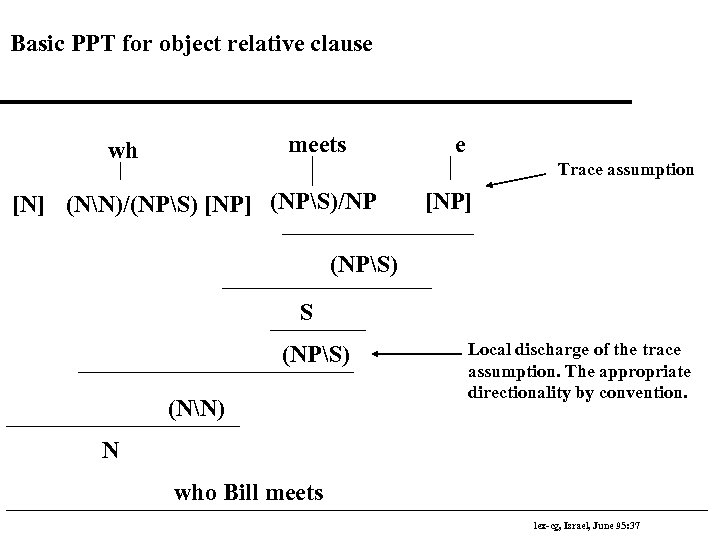Basic PPT for object relative clause meets wh e Trace assumption [N] (NN)/(NPS) [NP] (NPS)/NP [NP] (NPS) S (NPS) (NN) Local discharge of the trace assumption. The appropriate directionality by convention. N who Bill meets lex-cg, Israel, June 95: 37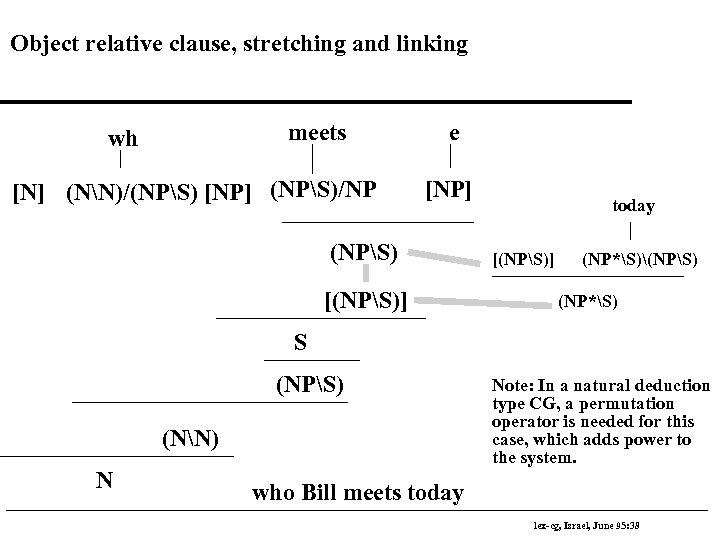Object relative clause, stretching and linking meets wh [N] (NN)/(NPS) [NP] (NPS)/NP e [NP] (NPS) [(NPS)] today [(NPS)] (NP*S)(NPS) (NP*S) S (NPS) (NN) N Note: In a natural deduction type CG, a permutation operator is needed for this case, which adds power to the system. who Bill meets today lex-cg, Israel, June 95: 38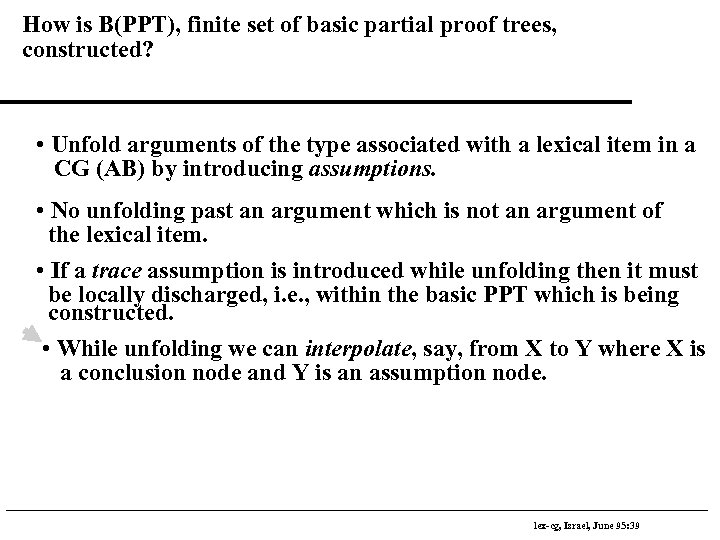How is B(PPT), finite set of basic partial proof trees, constructed? • Unfold arguments of the type associated with a lexical item in a CG (AB) by introducing assumptions. • No unfolding past an argument which is not an argument of the lexical item. • If a trace assumption is introduced while unfolding then it must be locally discharged, i. e. , within the basic PPT which is being constructed. • While unfolding we can interpolate, say, from X to Y where X is a conclusion node and Y is an assumption node. lex-cg, Israel, June 95: 39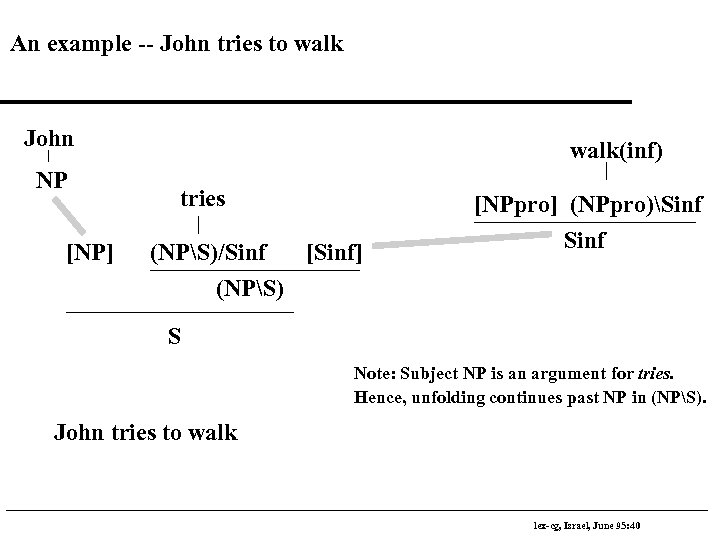An example -- John tries to walk John NP [NP] walk(inf) tries (NPS)/Sinf [Sinf] (NPS) [NPpro] (NPpro)Sinf S Note: Subject NP is an argument for tries. Hence, unfolding continues past NP in (NPS). John tries to walk lex-cg, Israel, June 95: 40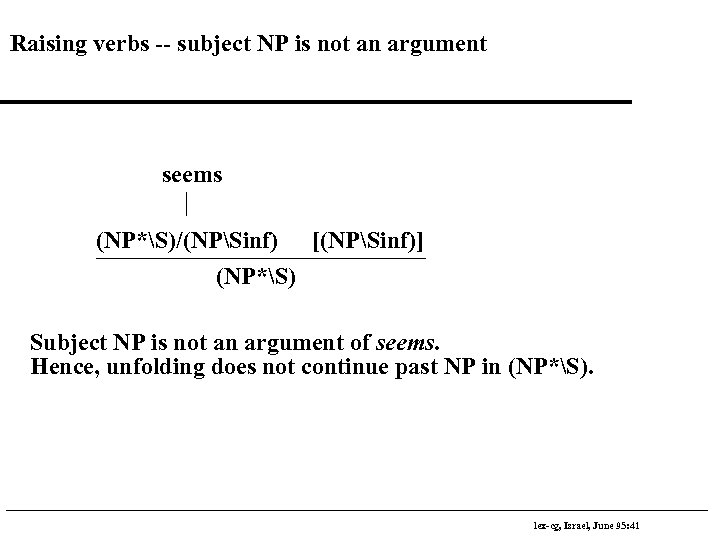Raising verbs -- subject NP is not an argument seems (NP*S)/(NPSinf) [(NPSinf)] (NP*S) Subject NP is not an argument of seems. Hence, unfolding does not continue past NP in (NP*S). lex-cg, Israel, June 95: 41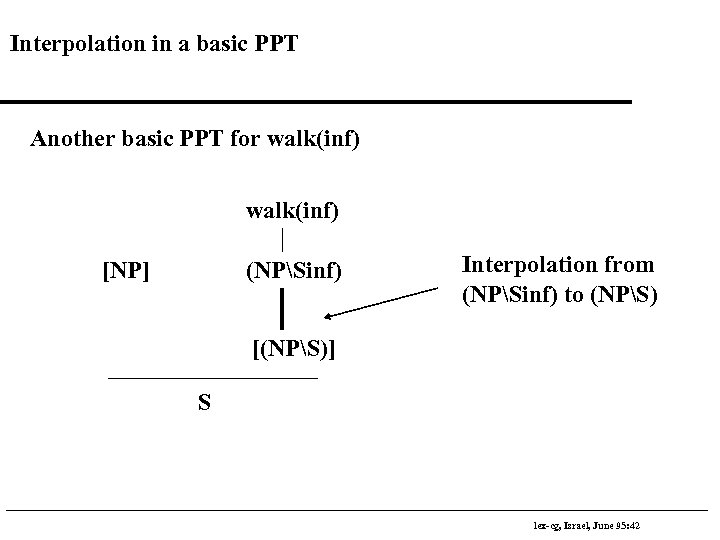Interpolation in a basic PPT Another basic PPT for walk(inf) [NP] (NPSinf) Interpolation from (NPSinf) to (NPS) [(NPS)] S lex-cg, Israel, June 95: 42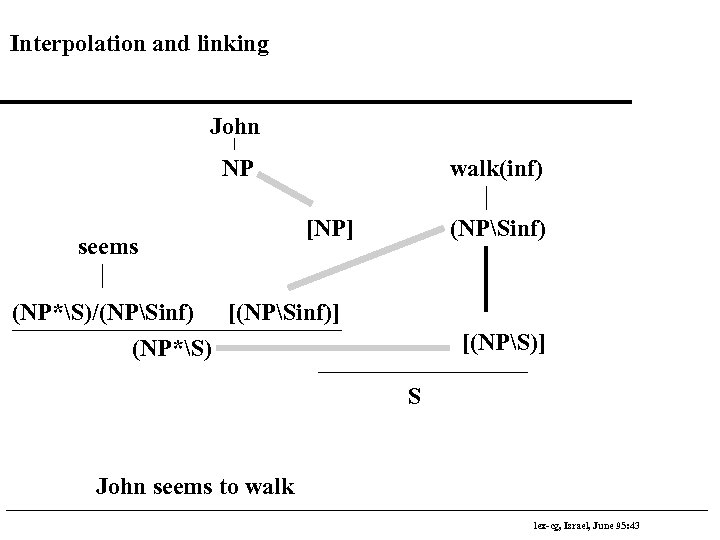Interpolation and linking John NP seems walk(inf) [NP] (NPSinf) (NP*S)/(NPSinf) [(NPSinf)] (NP*S) [(NPS)] S John seems to walk lex-cg, Israel, June 95: 43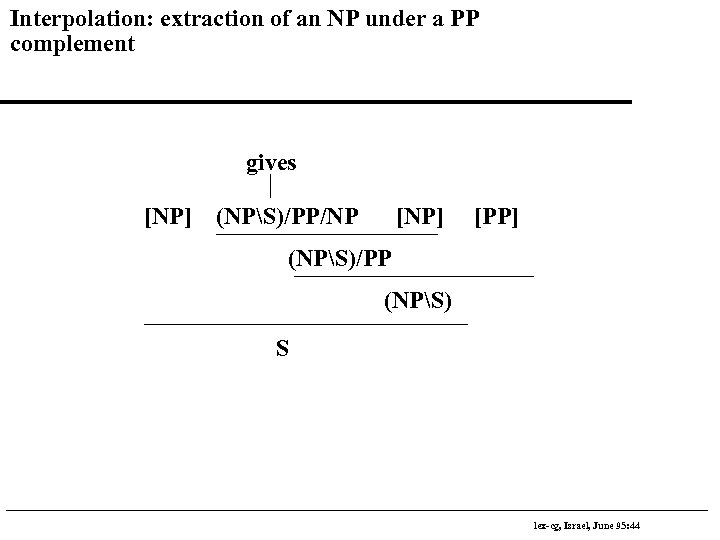Interpolation: extraction of an NP under a PP complement gives [NP] (NPS)/PP/NP [NP] [PP] (NPS)/PP (NPS) S lex-cg, Israel, June 95: 44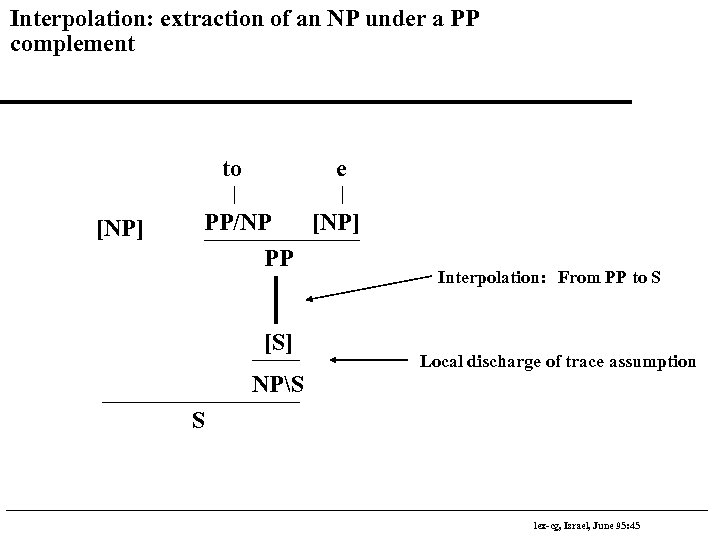Interpolation: extraction of an NP under a PP complement to [NP] e PP/NP [NP] PP [S] NPS Interpolation: From PP to S Local discharge of trace assumption S lex-cg, Israel, June 95: 45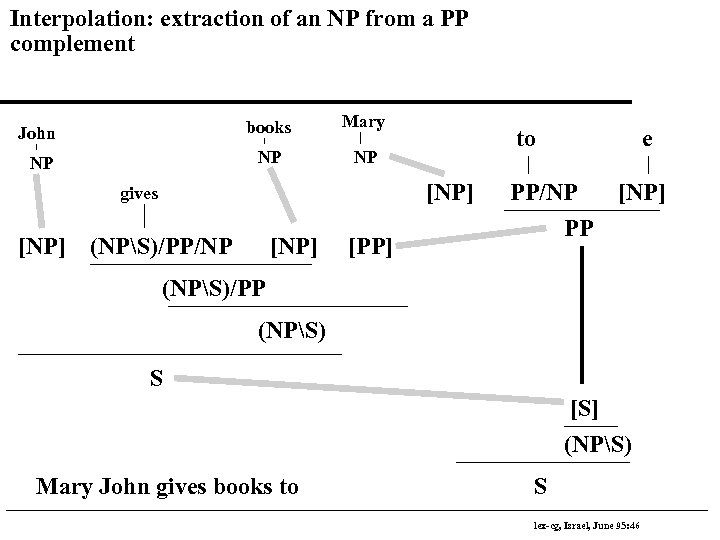Interpolation: extraction of an NP from a PP complement John books Mary NP NP NP [NP] gives [NP] to (NPS)/PP/NP [NP] [PP] e PP/NP PP [NP] (NPS)/PP (NPS) S [S] (NPS) Mary John gives books to S lex-cg, Israel, June 95: 46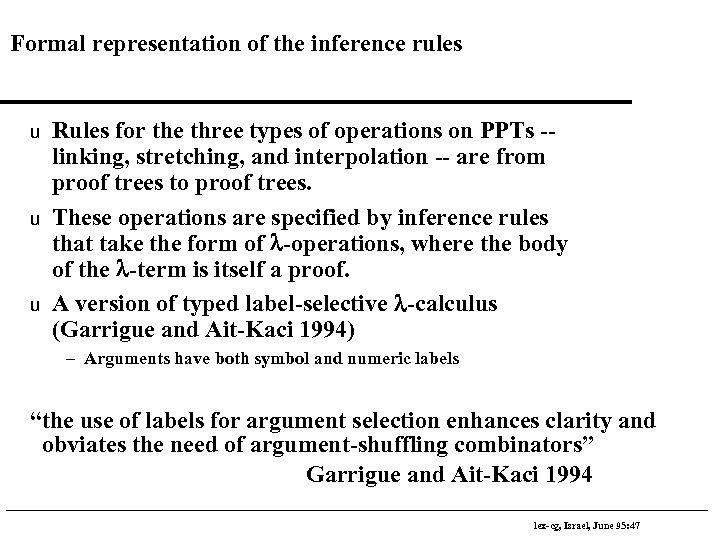Formal representation of the inference rules u u u Rules for the three types of operations on PPTs -linking, stretching, and interpolation -- are from proof trees to proof trees. These operations are specified by inference rules that take the form of l-operations, where the body of the l-term is itself a proof. A version of typed label-selective l-calculus (Garrigue and Ait-Kaci 1994) – Arguments have both symbol and numeric labels “the use of labels for argument selection enhances clarity and obviates the need of argument-shuffling combinators” Garrigue and Ait-Kaci 1994 lex-cg, Israel, June 95: 47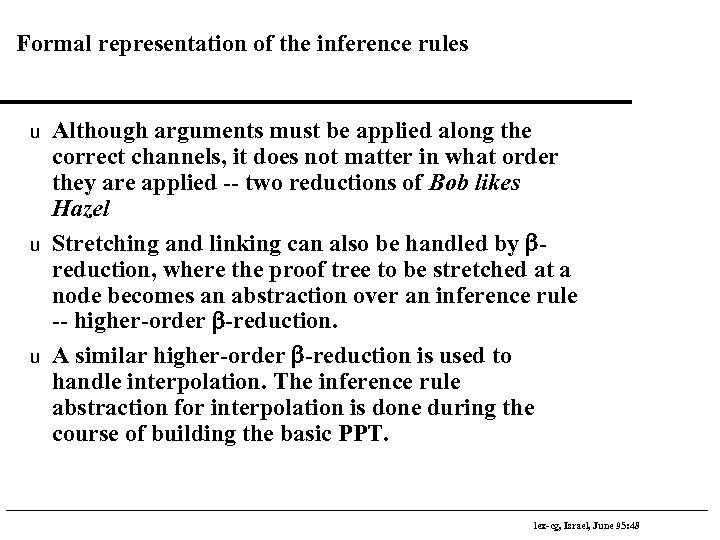Formal representation of the inference rules u u u Although arguments must be applied along the correct channels, it does not matter in what order they are applied -- two reductions of Bob likes Hazel Stretching and linking can also be handled by breduction, where the proof tree to be stretched at a node becomes an abstraction over an inference rule -- higher-order b-reduction. A similar higher-order b-reduction is used to handle interpolation. The inference rule abstraction for interpolation is done during the course of building the basic PPT. lex-cg, Israel, June 95: 48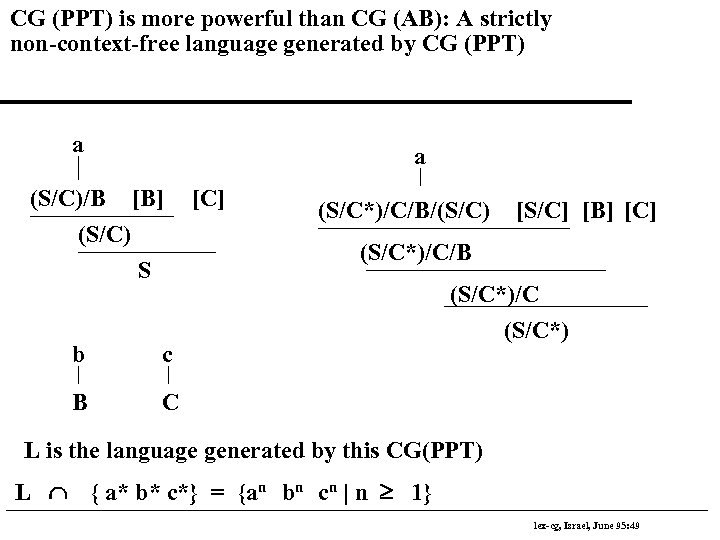CG (PPT) is more powerful than CG (AB): A strictly non-context-free language generated by CG (PPT) a a (S/C)/B [B] (S/C) S b (S/C*)/C/B/(S/C) [S/C] [B] [C] (S/C*)/C/B c B [C] (S/C*)/C (S/C*) C L is the language generated by this CG(PPT) L Ç { a* b* c*} = {an bn cn | n ³ 1} lex-cg, Israel, June 95: 49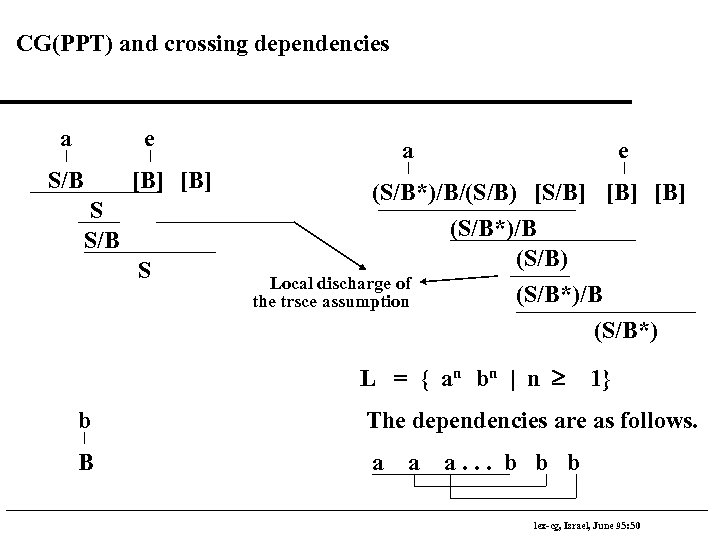CG(PPT) and crossing dependencies a e S/B [B] S S/B S a e (S/B*)/B/(S/B) [S/B] [B] (S/B*)/B (S/B) Local discharge of (S/B*)/B the trsce assumption (S/B*) L = { a n bn | n ³ 1} b The dependencies are as follows. B a a a. . . b b b lex-cg, Israel, June 95: 50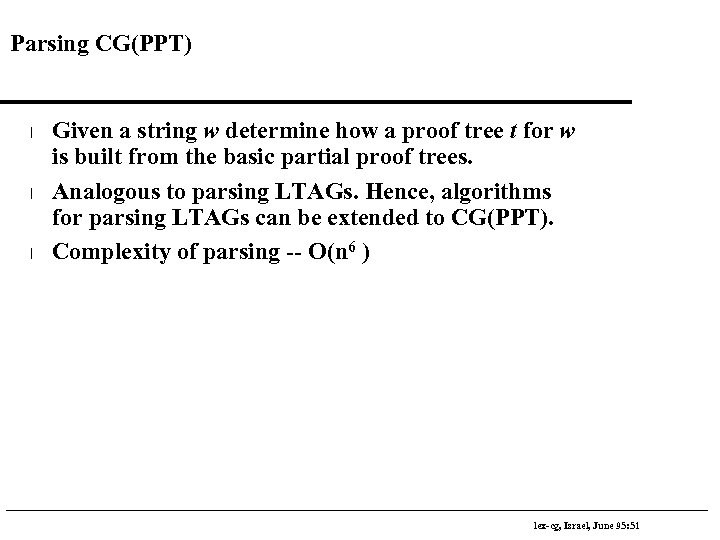Parsing CG(PPT) l l l Given a string w determine how a proof tree t for w is built from the basic partial proof trees. Analogous to parsing LTAGs. Hence, algorithms for parsing LTAGs can be extended to CG(PPT). Complexity of parsing -- O(n 6 ) lex-cg, Israel, June 95: 51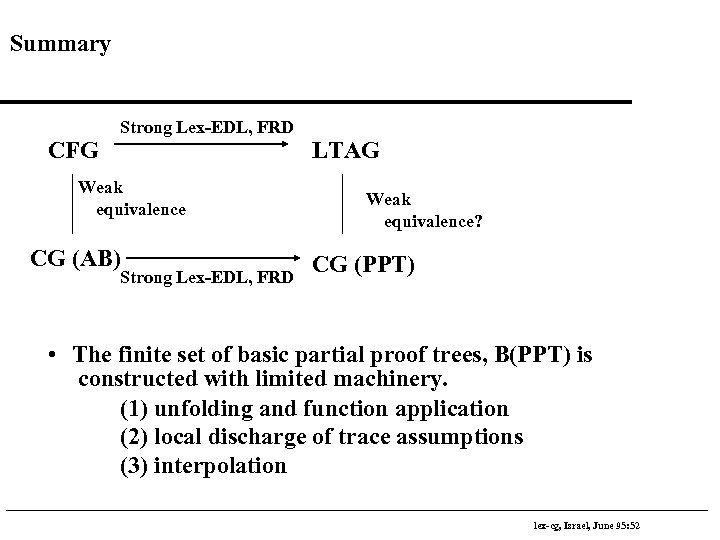Summary CFG Strong Lex-EDL, FRD Weak equivalence CG (AB) Strong Lex-EDL, FRD LTAG Weak equivalence? CG (PPT) • The finite set of basic partial proof trees, B(PPT) is constructed with limited machinery. (1) unfolding and function application (2) local discharge of trace assumptions (3) interpolation lex-cg, Israel, June 95: 52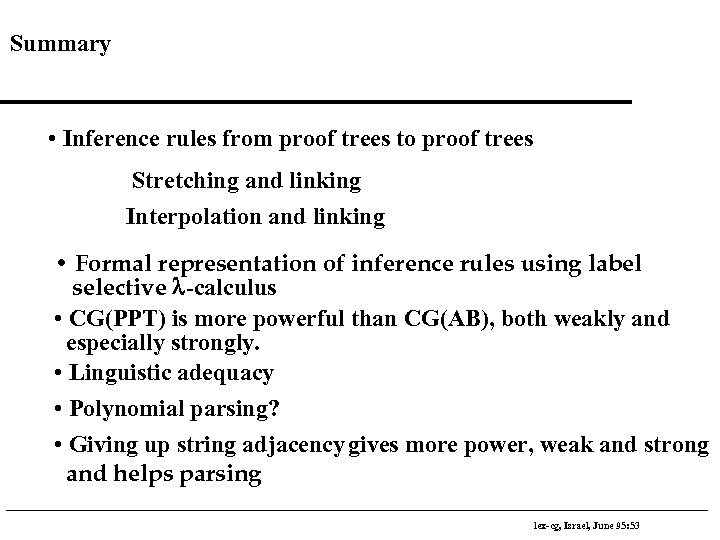Summary • Inference rules from proof trees to proof trees Stretching and linking Interpolation and linking • Formal representation of inference rules using label selective l-calculus • CG(PPT) is more powerful than CG(AB), both weakly and especially strongly. • Linguistic adequacy • Polynomial parsing? • Giving up string adjacency gives more power, weak and strong and helps parsing lex-cg, Israel, June 95: 53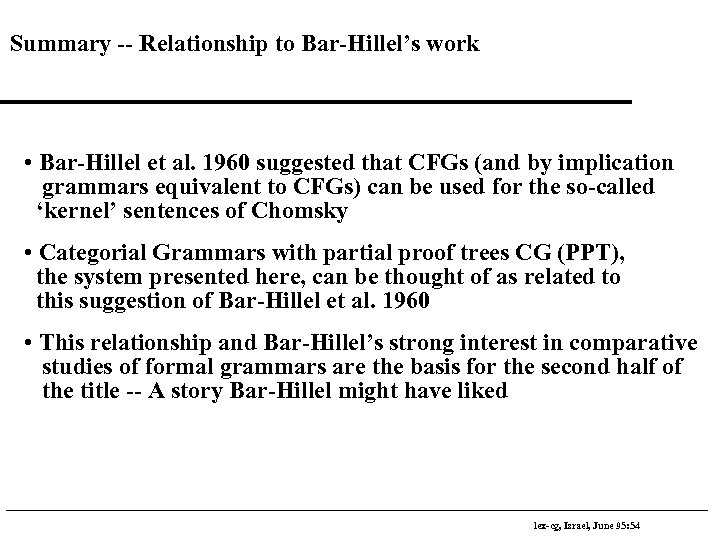Summary -- Relationship to Bar-Hillel’s work • Bar-Hillel et al. 1960 suggested that CFGs (and by implication grammars equivalent to CFGs) can be used for the so-called ‘kernel’ sentences of Chomsky • Categorial Grammars with partial proof trees CG (PPT), the system presented here, can be thought of as related to this suggestion of Bar-Hillel et al. 1960 • This relationship and Bar-Hillel’s strong interest in comparative studies of formal grammars are the basis for the second half of the title -- A story Bar-Hillel might have liked lex-cg, Israel, June 95: 54